Series convergence calculator

There are different ways of series convergence tesing. First of all one can just find series sum. If the value received is finite number, then the series is converged. For instance, because of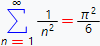the series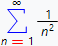is converged. If we wasn't able to find series sum, than one should use different methods for testing series convergence.

One of these methods is the ratio test, which can be written in following form: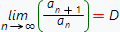here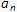and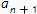is the n-th and (n+1) series members correspondingly, and convergence of the series is determined by the value of D: if D < 1 – series converged, if D > 1 – series diverged. If D = 1 – the ratio test is inconclusive and one should make additional researches.

As an example, test the convergence of the following series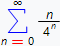by means of ratio test. First of all write out the expressions for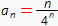and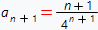. Then find corresponging limit: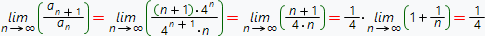Because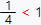, in concordance with ratio test, series converged.

Another method which is able to test series convergence is the root test, which can be written in the following form: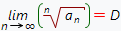hereis the n-th series member, and convergence of the series determined by the value of D in the way similar to ratio test: if D < 1 – series converged, if D > 1 – series diverged. If D = 1 – the root test is inconclusive and one should make additional researches.

As an example, test the convergence of the following series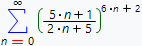by means of root test. First of all, write out the expression for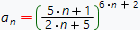. Then find the corresponding limit: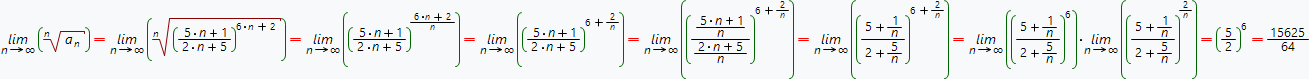Because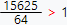, in accordance with root test, series diverged.

It should be noted, that along with methods listed above, there are also exist another series convergence testing methods such as integral test, Raabe test and ect.

Our online calculator, build on Wolfram Alpha system is able to test convergence of different series. It should be noted, that if the calculator finds sum of the series and this value is the finity number, than this series converged. In the opposite case, one should pay the attention to the «Series convergence test» pod.

Below listed the explanation of possible values of «Series convergence test» pod:

The «Series convergence test» pod value Explanation
By the harmonic series test, the series diverges. Then the series was compared with harmonic one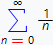, initial series was recognized as diverged.
The ratio test is inconclusive. The application of ratio test was not able to give understanding of series convergence because the value of corresponding limit equals to 1 (see above).
The root test is inconclusive. The application of root test was not able to give understanding of series convergence because the value of corresponding limit equals to 1 (see above).
By the comparison test, the series converges. When the comparison test was applied to the series, it was recognized as diverged one.
By the ratio test, the series converges. The ratio test was able to determined the convergence of the series
By the limit test, the series diverges. Because of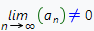, or the mentioned limit does not exist, the series was recognized as diverged one.
Series convergence calculator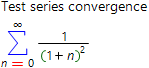Input series which convergence you want to test